## Homework 2

1. Show that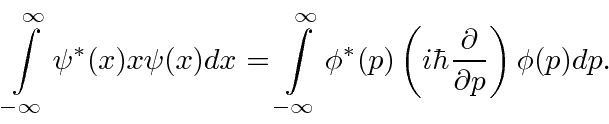Remember that the wave functions go to zero at infinity.

2. Directly calculate the the RMS uncertainty infor the stateby computing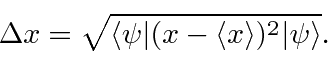3. Calculatefor the state in the previous problem. Use this to calculatein a similar way to thecalculation.

4. Calculate the commutator.

5. Consider the functions of one anglewithand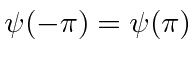. Show that the angular momentum operatorhas real expectation values.

6. A particle is in the first excited state of a box of length. What is that state? Now one wall of the box is suddenly moved outward so that the new box has length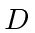. What is the probability for the particle to be in the ground state of the new box? What is the probability for the particle to be in the first excited state of the new box? You may find it useful to know that7. A particle is initially in the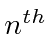eigenstate of a box of length L. Suddenly the walls of the box are completely removed. Calculate the probability to find that the particle has momentum betweenand. Is energy conserved?

8. A particle is in a box with solid walls at. The state atis constantforand theeverywhere else. Write this state as a sum of energy eigenstates of the particle in a box. Writein terms of the energy eigenstates. Write the state atas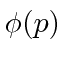. Would it be correct (and why) to useto compute?

9. The wave function for a particle is initially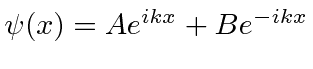. What is the probability flux?

10. Prove that the parity operator defined byis a hermitian operator and find its possible eigenvalues.

Jim Branson 2013-04-22